### Home > A2C > Chapter 4 > Lesson 4.2.1 > Problem4-77

4-77.
1. People who live in isolated or rural areas often have their own tanks that hold gas to run appliances like stoves, washers, and water heaters. Some of these tanks are made in the shape of a cylinder with two hemispheres on the ends, as shown in the picture at right. (A hemisphere is half of a sphere, and the volume of a sphere is found by using V = 4/3πr3.)

2. The Inland Propane Gas Tank Company wants to make tanks with this shape, but offer different models in different sizes. The cylindrical portion of each of the tanks will be 4 meters long. However, the radius r will vary among the different models. Homework Help ✎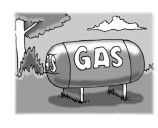1. One of their tanks has a radius of 1 meter. What is its volume?

2. When the radius doubles (to 2 meters), will the volume double? Explain. Then figure out the volume of the larger tank with r = 2 m.

3. Write an equation that will let Inland Propane Gas Tank Company determine the volume of a tank with any size radius.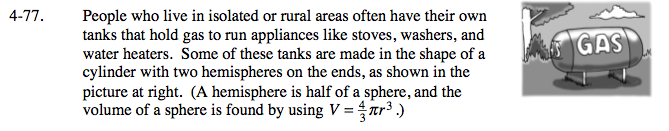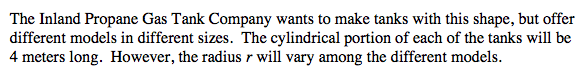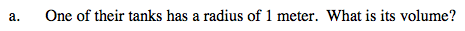Substitute 1 for r in the sphere and cylinder equations.

V = 4π

$\textit{V}=\frac{4}{3}\pi$

$\textit{V}=4\pi + \frac{4}{3}\pi$

$\frac{16}{3}\pi \approx 16.755 m^3$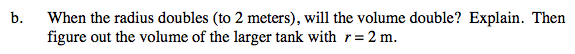Remember that the radius is being cubed.

The radius will not double because of the r, r2, r3 relationship.

Now, see part (a).

$\textit{V}=16\pi + \frac{32}{3}\pi$

The tank will be 83.776m3.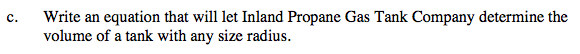Remember that the total is the combination of a sphere and a cylinder.

$\textit{V}=\frac{4}{3}\pi\textit{r}^{3}+4\pi\textit{r}^{2}$